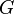# Gravitational constant facts for kids

Kids Encyclopedia Facts

The gravitational constant, called$G$ in physics equations, is an empirical physical constant. It is used to show the force between two objects caused by gravity. The gravitational constant appears in Isaac Newton's universal law of gravitation.$G$ is about 6.67430×10−11 N⋅m2/kg2, and is denoted by letter$G$.

It usually appears in Sir Isaac Newton's law of universal gravitation, and in Albert Einstein's general theory of relativity. It is also known as the universal gravitational constant, Newton's constant, and colloquially as Big G. It should not be confused with "small g" (g), which is the local gravitational field of the Earth (equivalent to the free-fall acceleration).

## Images for kidsGravitational constant Facts for Kids. Kiddle Encyclopedia.